# Collision theory and Activation Energy Temperature and Activation

• Slides: 13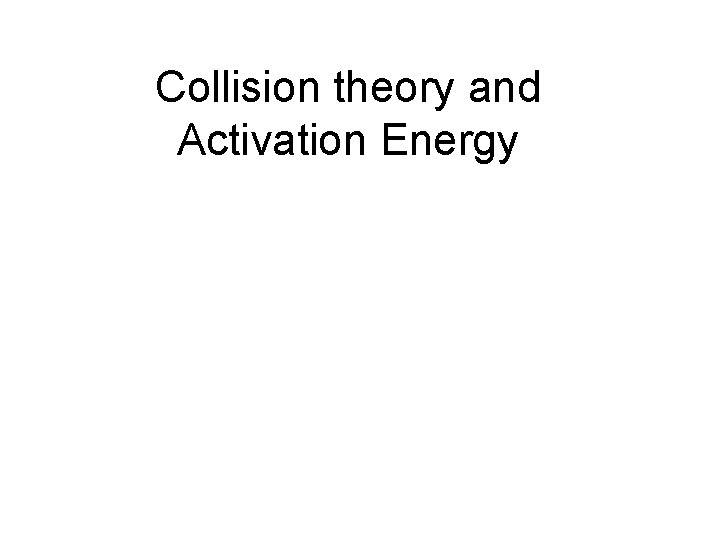Collision theory and Activation Energy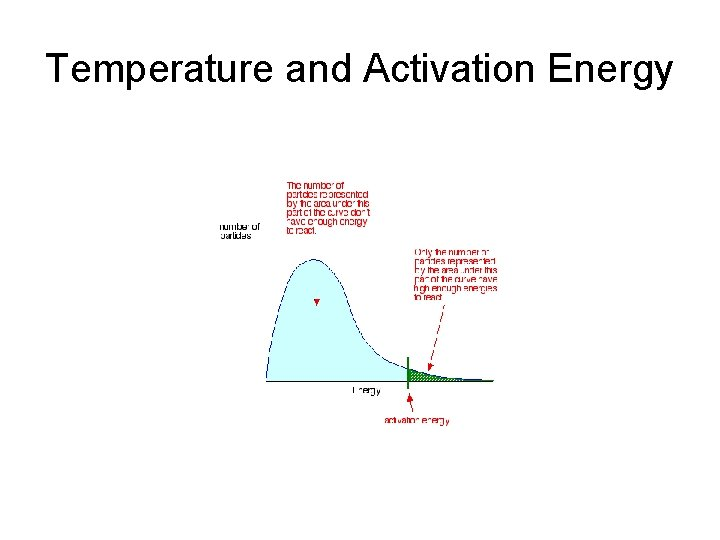Temperature and Activation EnergyTemperature and in EaArrhenius Equation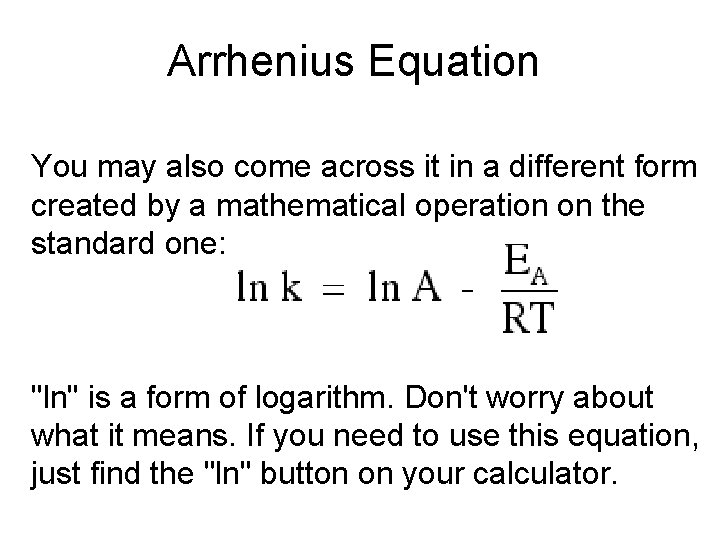Arrhenius Equation You may also come across it in a different form created by a mathematical operation on the standard one: "ln" is a form of logarithm. Don't worry about what it means. If you need to use this equation, just find the "ln" button on your calculator.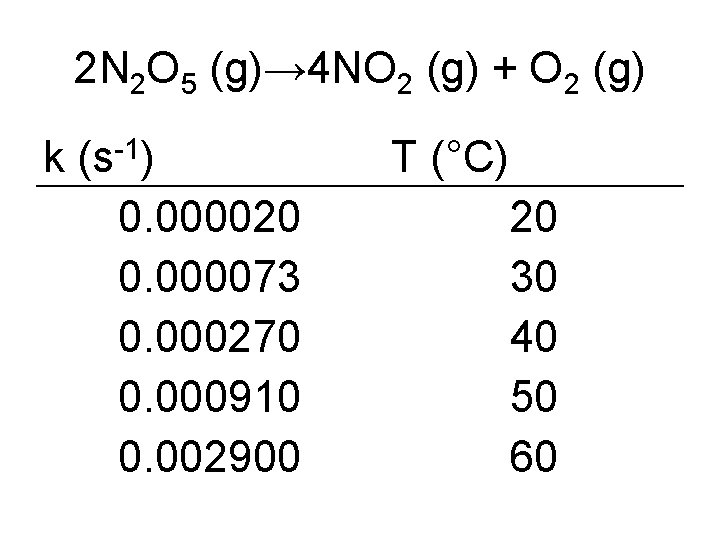2 N 2 O 5 (g)→ 4 NO 2 (g) + O 2 (g) -1 k (s ) 0. 000020 0. 000073 0. 000270 0. 000910 0. 002900 T (°C) 20 30 40 50 60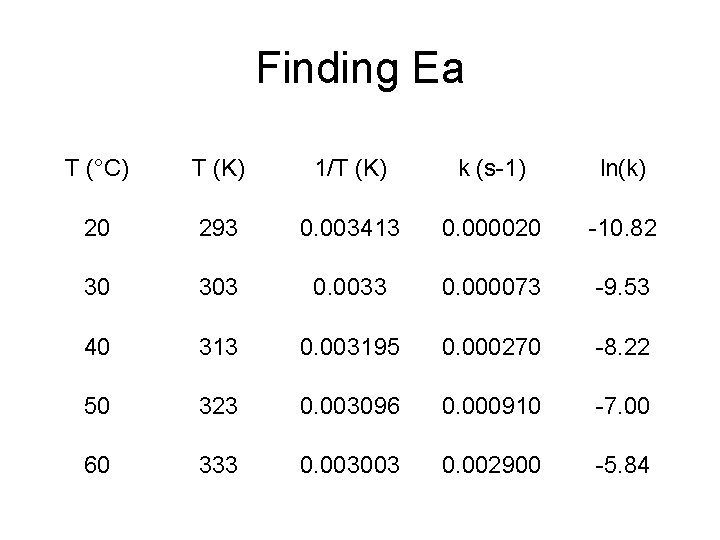Finding Ea T (°C) T (K) 1/T (K) k (s-1) ln(k) 20 293 0. 003413 0. 000020 -10. 82 30 303 0. 0033 0. 000073 -9. 53 40 313 0. 003195 0. 000270 -8. 22 50 323 0. 003096 0. 000910 -7. 00 60 333 0. 003003 0. 002900 -5. 84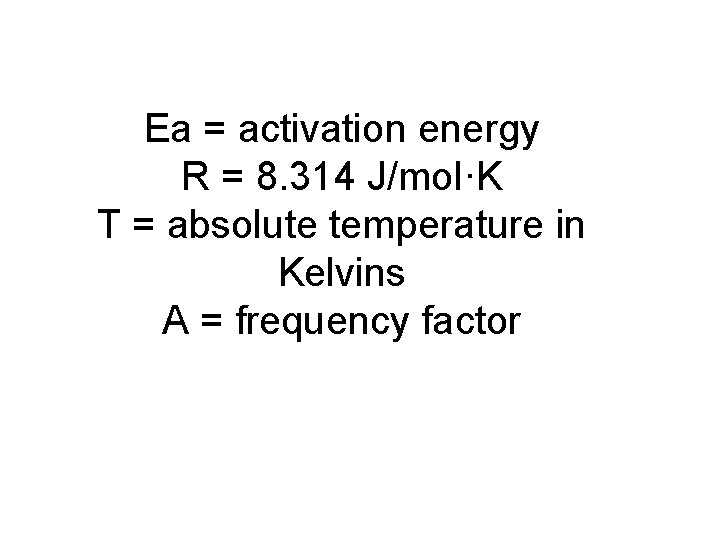Ea = activation energy R = 8. 314 J/mol·K T = absolute temperature in Kelvins A = frequency factor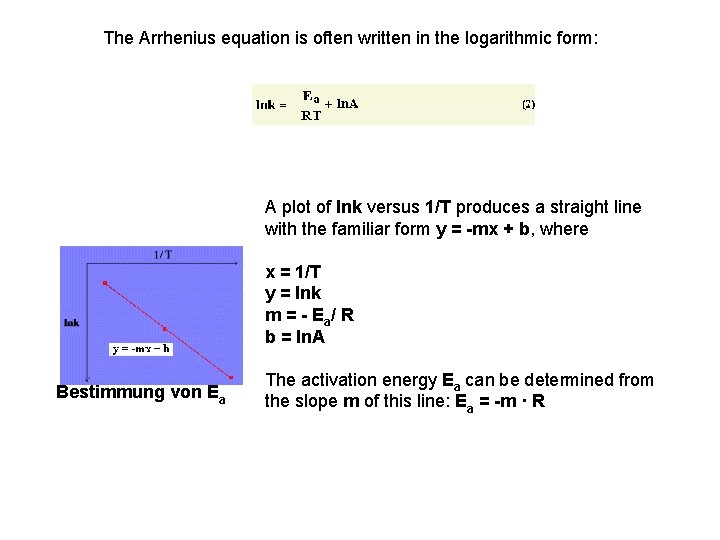The Arrhenius equation is often written in the logarithmic form: A plot of lnk versus 1/T produces a straight line with the familiar form y = -mx + b, where x = 1/T y = lnk m=-E /R b = ln. A a Bestimmung von Ea The activation energy Ea can be determined from the slope m of this line: Ea = -m · R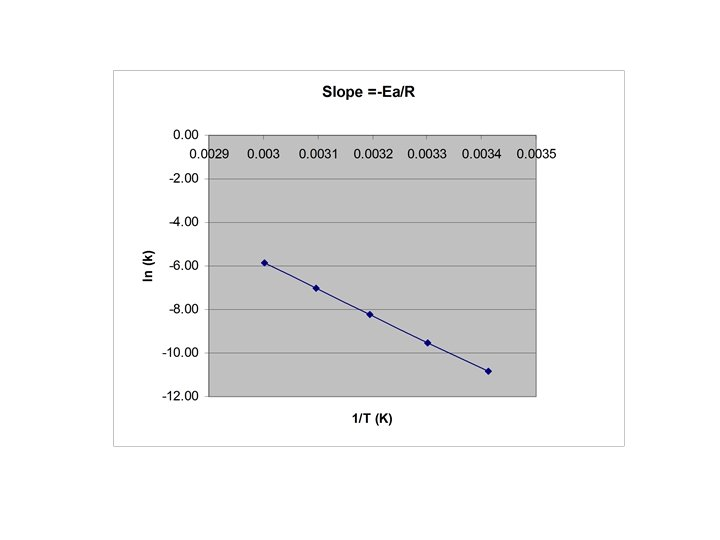Ea=-R(slope) • Slope =Δln(k)/Δ(1/T) • Ea=-(8. 3145 J/K*mol)(-12000 K) • =100, 000 J/mol